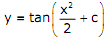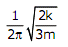# Consider  the  differential  equation The  general  solution  with constant c is

1.

2.

3.

4.

4Explanation :
No Explanation available for this question

# An unbiased coin is tossed five times. The outcome of each toss is either a head  or a tail. The probability of getting at least one head is

1.  1/32

2.  13/32

3.  16/32

4.  31/32

4

31/32

Explanation :
No Explanation available for this question

# A mass of 1kg is attached to two identical springs each with stiffness k = 20kN/m as shown in the figure. Under frictionless condition, the natural frequency of the  system in Hz is close to

1.  32

2.  23

3.  16

4.  11

4

32

Explanation :
No Explanation available for this question

# The shear  strength of a sheet metal  is 300MPa. The blanking  force  required  to produce a blank of 100mm diameter from a 1.5 mm thick sheet is close to

1.  45kN

2.  70kN

3.  141kN

4.  3500kN

4

141kN

Explanation :
No Explanation available for this question

# The  ratios  of  the  laminar  hydrodynamic  boundary  layer  thickness  to  thermal  boundary layer thickness of flows of two fluids P and Q on a flat plate are 1/2  and 2 respectively. The Reynolds number based on the plate length for both the flows 104. The  Prandtl  and Nusselt numbers  for  P  are 1/8 and 35  respectively.  The Prandtl and Nusselt numbers for Q are respectively

1.  8 and 140

2.  8 and 70

3.  4 and 70

4.  4 and 35

4

8 and 140

Explanation :
No Explanation available for this question

# The crank  radius of a single–cylinder  I. C. engine  is 60mm and  the diameter of  the cylinder is 80mm. The swept volume of the cylinder in cm3 is

1.  48

2.  96

3.  302

4.  603

4

603

Explanation :
No Explanation available for this question

# A  pump  handling  a  liquid  raises  its  pressure  from  1  bar  to  30  bar.  Take  the density  of  the  liquid  as  990 kg/m3. The  isentropic  specific  work  done  by  the pump in kJ/kg is

1.  0.10

2.  0.30

3.  2.50

4.  2.93

4

2.93

Explanation :
No Explanation available for this question

# A spherical steel ball of 12mm diameter is initially at 1000K. It is slowly cooled in a  surrounding of 300K. The heat  transfer  coefficient between  the steel ball and the surrounding  is 5W/m2K. The thermal conductivity of steel is 20W /mK . The temperature difference between the centre and the surface of the steel ball is

1.  Large  because  conduction  resistance  is  far  higher  than  the  convective resistance

2.  Large because conduction resistance is far less than the convective resistance

3.  Small  because  conduction  resistance  is  far  higher  than  the  convective resistance

4.  Small because conduction resistance is far less than the convective resistance

4

Small because conduction resistance is far less than the convective resistance

Explanation :
No Explanation available for this question

# An ideal Brayton cycle, operating between the pressure limits of 1 bar and 6 bar, has  minimum  and  maximum  temperatures  of  300K  and  1500K.  The  ratio  of specific heats of the working  fluid  is 1.4. The approximate  final temperatures  in Kelvin at the end of the compression and expansion processes are respectively

1.  500 and 900

2.  900 and 500

3.  500 and 500

4.  900 and 900

4

500 and 900

Explanation :
No Explanation available for this question

# A disc of mass m is attached to a spring of stiffness k as shown in the figure. The disc  rolls  without  slipping  on  a  horizontal  surface.  The  natural  frequency  of vibration of the system is

1.

2.

3.

4.

4